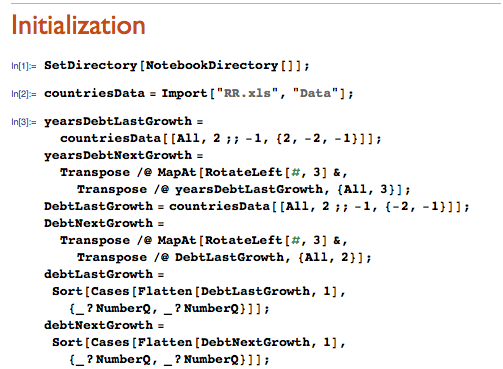Browse by Topic
Related Topics

# Is High Debt Bad for Economic Growth?

Recent events have brought to light that a famous research paper by noted economists Carmen Reinhart and Kenneth Rogoff published in the 2010 National Bureau of Economic Research working paper series contained serious programming, methodological, and statistical errors. Their paper claimed to show that there is a sharp decline in GDP whenever an economy reaches the 90% debt level. Policy makers at the European Commission and in Washington paid heed to these results and formulated real policies such as “debt stabilization” in Europe and the sequester in the USA that have led to sustained high unemployment and weakened economies. But subsequent analyses have shown that no such 90% cliff exists.

I will show that such errors are quite unlikely to occur in Mathematica because of its explicit comprehensive functional programming style, which not only makes coding simpler and quicker, but allows for rapid sensitivity tests for robustness. The current debacle came to light after Reinhart and Rogoff (R&R) finally released their dataset, which was then analyzed in detail by Thomas Herndon, Michael Ash, and Robert Pollin (HAP 2013) from the University of Massachusetts Amherst. I am not going to replicate their results, but rather show how similar analyses can be done in Mathematica while also addressing related problems of causality and conditional probability estimation that were not addressed in the initial publication.

### Data Description

The data for the original investigation can be obtained here. I have adapted it to contain the important variables of Debt/GDP and Real GDP (RGDP) Growth that are the basis of analysis in all the papers discussed above. The one main area of difference is that I use the three-year RGDP Growth as a variable rather than the usual one-year approach. This is because any real measure of policy determination for the \$16 trillion US economy would not look just one year into the future but three years to properly assess its effects. This is important to keep in mind because my RGDP Growth figures will appear to be approximately three times that in R&R’s paper.

These two variables, Debt/GDP and three-year RGDP Growth, are the only additional columns that I have made to the original R&R Excel workbook, RR.xls. I have also included all the historical data in order to include important data points such as the 1930s and the Great Depression as well as the financial crises in the 1870s, 1890s, and immediately before WWI, as opposed to most studies that only looked at data after 1945. These data points were especially important for our current economic situation because they were significant financial crises in which monetary policy was largely irrelevant, exactly as we have now. For additional details about the data, see the footnote at the bottom of the blog post.

### Data Import and Analysis

I imported R&R’s data into Mathematica from their Excel spreadsheet, giving an array of data about the economic variables of debt and GDP growth for each country: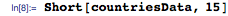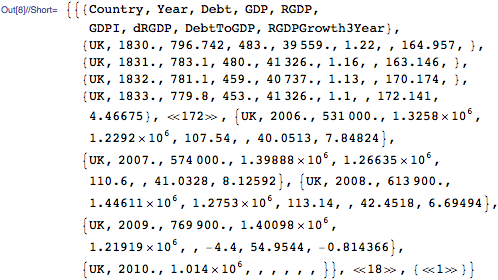These are the countries whose economic variables were studied: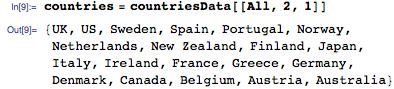In this analysis, I am most interested in the Debt to GDP ratio and three-year RGDP Growth variables. This differs from the original R&R analysis, where they concentrated on one-year RGDP Growth. This is to allow for three-year prediction intervals rather than limiting the analysis to just one year ahead. Let’s take a look at these two variables for all the countries that are in our data sheet.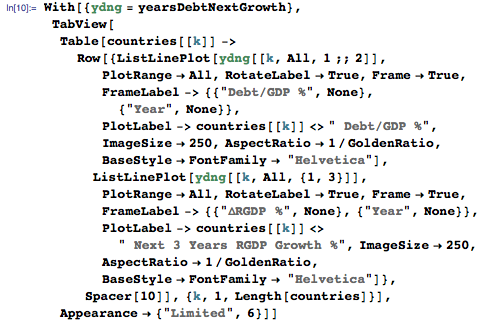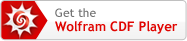Using the conclusions from R&R’s original 2010 paper as well as HAP’s corrected calculations based on R&R’s methodology, which are described in Table 3 of HAP’s recent review article, I find the following comparative results represented graphically. The blue bars represent R&R’s original results. The green bars show HAP’s corrected results.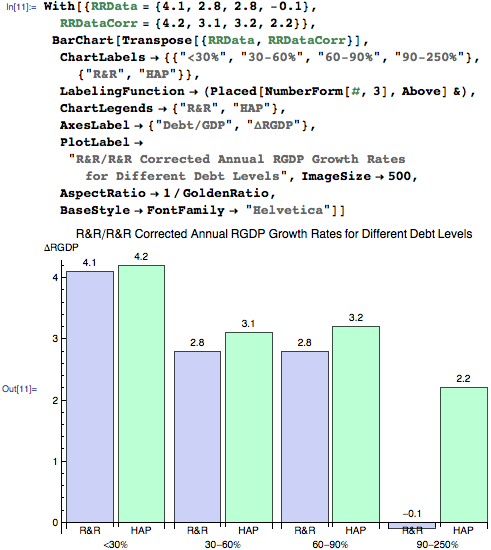Notice the HAP results revise upward by 2.3% R&R’s negative growth rate of -0.1% for countries whose debt exceeds 90%. Furthermore, the difference in growth rates from the 60–90% cohort to the >90% group is not statistically significant.

Suppose you accept the approach adopted by R&R that debt is a driving factor detrimentally affecting growth, then you should be able to test that notion by regressing growth upon debt and studying the consequences. If that analysis is performed, then you get the following linear regression between Y:RGDP % annual Growth and X:nominal Debt/GDP ratio, Y = -0.0229 X + 3.8998, as described by Paul Krugman in his blog post, which shows that each percentage increase in Debt/GDP reduces annual RGDP by merely .0229% not including the intercept constant. I can adopt the same argument that Krugman and Brad DeLong make that even if this relation is causal running from high debt to slower future growth, then this still does not warrant cuts in government spending now. The effects of government spending on GDP growth are called the fiscal multiplier, and recent estimates of this by the IMF Fiscal Monitor estimate the multiplier to be about 1.5. This means that every dollar spent by the government leads to \$1.5 GDP growth.

So should we cut spending now, resulting in lost jobs and reduced growth, in order to avoid a possible growth decline a decade later? Using estimates of fiscal multiplier, current US marginal tax rate, and linear regression between real GDP annual growth rates and the nominal Debt/GDP ratios, I visualize the effect on GDP a decade later.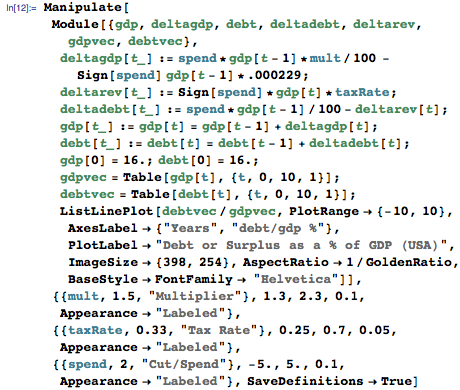From the interactive chart above, you can see that a reduction of 2% GDP now results in a statistically insignificant 0.23% GDP growth 10 years from now! And conversely, if we boost government spending by 2% of GDP now, the net effect will be to lower GDP by only 0.23% a decade later.

A different type of analysis would be to look at individual countries and see whether regressions of RGDP Growth against Debt/GDP show a downward trend in growth for higher levels of debt. The following tab display gives these regressions for all the different countries: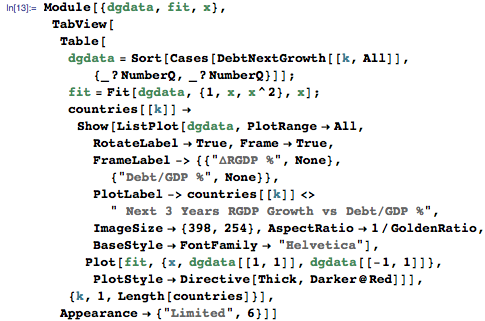Looking at individual countries, I can observe the slope of the regression at very high levels of debt to see which way it is trending, and I find that there are 10 countries with a slight trend up and 10 countries with a slight trend down. But the real observation is that for many, the slope at 90% Debt/GDP is not significantly different from zero, showing that changes in debt at that level have little effect on GDP. Seven countries are flat or indifferent at the 90% mark, five are trending down, three are trending up, and five lack the relevant data, probably because they have either never reached this threshold or did so when other reliable data was not available. So out of the 15 countries I can study at the 90% debt level, 10 or 75% of them are either no worse off or appear to be growing, while 1/3 appear to be getting worse. This does not appear to support the notion that only calamity can result when we cross the magical 90% Debt/GDP threshold.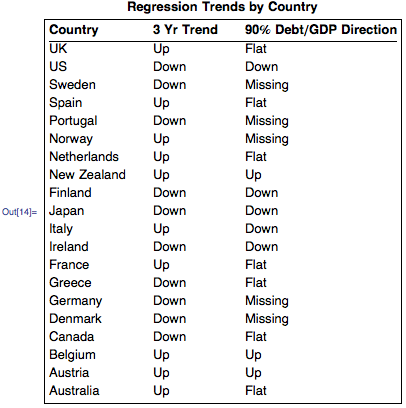The real popularity of R&R’s paper rested on their graph of mean growth rates at different levels of debt, which showed a sudden cliff at the 90% Debt/GDP level, dropping to -0.1% RGDP Growth. No one was able to replicate this result even when they used R&R’s previous data from their book This Time Is Different. In fact, most other researchers insisted that the obviously better approach was to use medians rather than means when doing statistical analysis. When R&R adopted this scheme themselves, the so-called growth cliff also disappeared, but remarkably they did not infer from this that their analysis was not robust or that the distributions were inconsistent. When eventually three years later they released their data, it was shown that this result was an illusory byproduct of an Excel coding error, and that when their own analysis was done successfully, the discrepancy disappeared.

In the analysis below I have not used their debatable weighting scheme, but instead have given equal weight to each data point {Debt/GDP, 3 Yr RGDP Growth} for each country, which should be the default case. Also I am looking at three-year growth rates, not one-year increments. I can see below that there is no magical growth cliff at the 90% Debt/GDP level, and that if there is any significant result, it appears that some high-debt countries in the 60–90% range showed high mean growth while the median performance was slightly poorer than for lower debt levels. This indicates that growth responses to large debt differed considerably between countries, with some responding positively, which does not fit in with the R&R notion that large debt is always bad.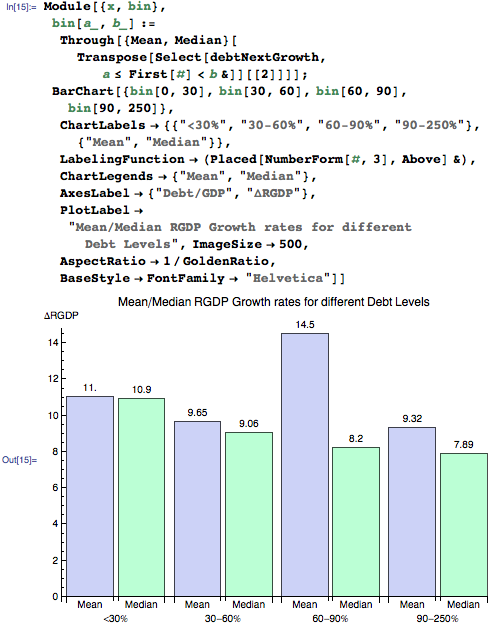A more sophisticated analysis can be done using conditional probability estimates by looking at the distributions associated with the data. Here I look at the overall conditional probability of RDGP Growth given Debt > 90% and compare it with the case when Debt lies between 60 and 90%. I see that their CDF structures are remarkably similar, suggesting similar probability distributions. This is also inconsistent with the R&R results, which suggest a different regime with higher levels of debt.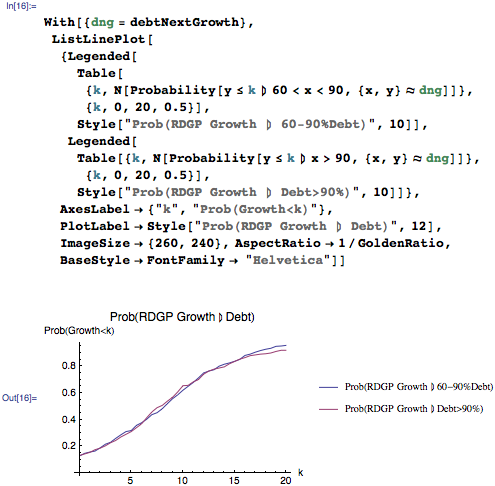Another important issue with the R&R approach is that they implicitly argue that high debt causes slow growth, but it is also possible that the causation runs the other way and that slow growth with a lack of revenues drives up the debt.

The above instances of Italy and Japan show that this is at least as likely as the other possibility. I can investigate this further by putting all the data together and doing regressions for the last three years and the next three years of real GDP growth.

If it is the case that high debt causes exceptionally slow growth, then this should show up as a significant regression at the 90% level on future growth, whereas if it is the other way around, I should see significant regression on past growth.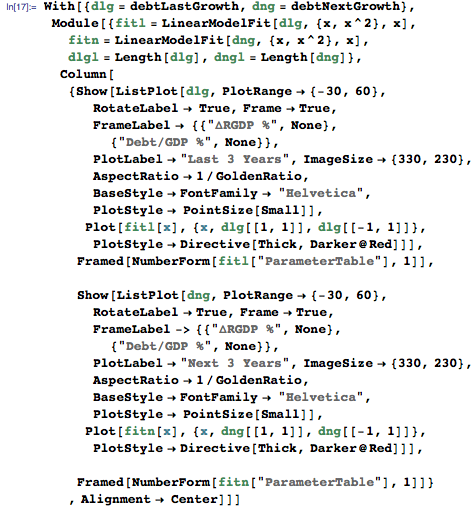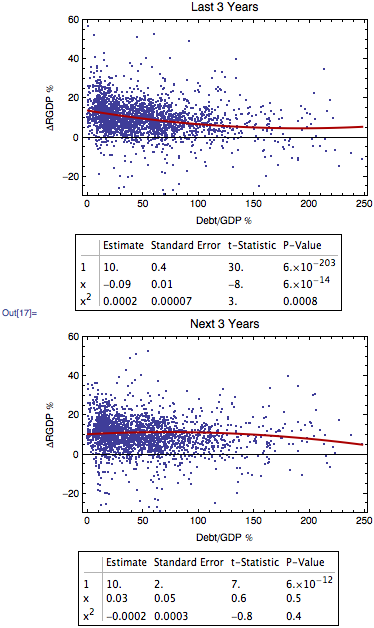From the regression and parameter tables above, I find a very small statistically significant positive slope of the trend line for future growth at 90% Debt and a corresponding mildly negative slope of the trend line on past growth, but which is not statistically significant. So the slope of the regression curve appears to be flat in both directions, and hence causation cannot be determined conclusively by regression approaches.

One important criticism of the R&R approach, especially after the work of HAP, is that they never explicitly identified their weighting methodology of country mean growth rates for different debt bins. This has been described above, and it raises the question of how different weighting mechanisms might work. Suppose for instance that I sort the data according to their debt levels and give higher weightings to those data points that have higher debt levels on a sliding linear scale, starting at 1 for equal weighting up to 11 times the weight for the highest levels of debt when compared to the lowest levels of debt. I can see that this increases the significance of debt on future growth, but only at very high levels of distortionary weighting.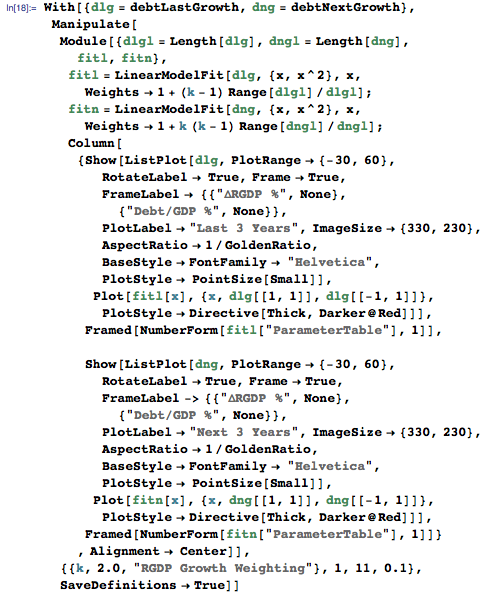Another approach would be to look more closely at the distributional differences between the four Debt/GDP bins that R&R use. If I use their four bins of debt levels and look at the overall histograms, I see that while the first two levels are comparable, the last two are different from the first two but are themselves comparable. This was also suggested from the above probability analysis.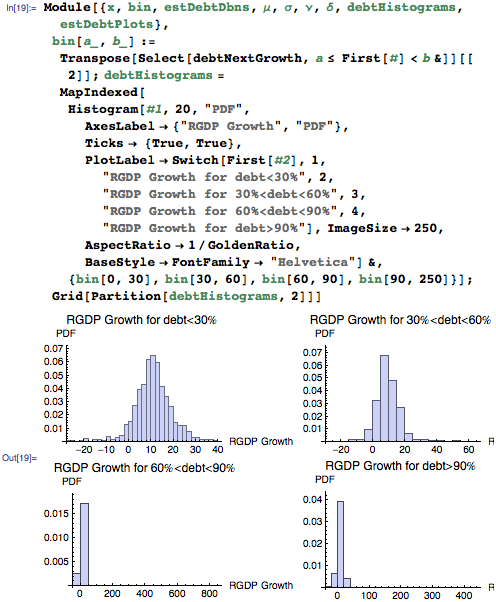Using the sparse Mathematica code given above, I show that high levels of debt are not a necessary barrier to GDP growth, that there are at least as many countries doing well with high debt as are doing badly, and that it is at least as likely that the causation runs the other way, where slow growth is causing high debt so that we are damaging the economy by cutting back spending now for fear of invoking the wrath of the debt demons. Since these high-level analyses can be carried out conveniently in Mathematica, the errors made by R&R using Excel could easily have been avoided.

### Footnote—Data Description Details

Because each spreadsheet had similar variables in different columns for different countries, the additional computations for Debt/GDP and three-year Real GDP Growth were done using simple Excel assignments. All variables are given in terms of percentages. The Debt/GDP variable was determined by taking ratios of corresponding debt and GDP values in the same year for the same country. If there were multiple debt and GDP columns starting at different times, as often occurred when countries switched currency regimes (for instance, when European countries all went onto the euro), then those new ratios were utilized at the later starting dates. The three-year Real GDP Growth variable was determined by looking at the percentage rate of change of RGDP over three-year periods, and again when there were multiple RGDP columns starting at different times, the new RGDP Growth series was adopted at the later starting dates.